Animal trajectory analysis with trajr

Introduction

The trajr R package is a toolkit for the numerical characterisation and analysis of the trajectories of moving animals. Trajectory analysis is applicable in fields as diverse as optimal foraging theory, migration, and behavioural mimicry (e.g. for verifying similarities in locomotion). A trajectory is simply a record of the path followed by a moving animal. Trajr operates on trajectories in the form of a series of locations (as x, y coordinates) with times. Trajectories may be obtained by any method which provides this information, including manual tracking, radio telemetry, GPS tracking, and motion tracking from videos.

The goal of this package (and this document) is to aid biological researchers, who may not have extensive experience with R, to analyse trajectories without being handicapped by a limited knowledge of R or programming. However, a basic understanding of R is required.

Within this document, the plots are generated by running the code examples immediately before them. For that reason, most code examples start with a call to TrajGenerate, which generates a random trajectory to be plotted. These calls would not occur in an analysis of real animal trajectories.

If you use trajr in your publications, please cite McLean DJ, Skowron Volponi MA. trajr: An R package for characterisation of animal trajectories. Ethology. 2018;00:1–9. https://doi.org/10.1111/eth.12739.

Installation and setup

Before using any R package, it is first necessary to install and load it. To install the latest released version of trajr, simply run:

install.packages("trajr")

To install the latest development version, which is available on GitHub, run:

install.packages("devtools")
devtools::install_github("JimMcL/trajr")

Once the package has been installed, you must load it, then it’s ready for use:

library("trajr")

Packages only need to be installed once, but they must be loaded in every session before they can be used.

Once the trajr package has been installed and loaded, you have access to the set of R functions it provides. All trajr functions (other than the generic functions plot, lines and points) start with the prefix Traj. Several of the functions provided by trajr do not provide additional analytic functionality, rather they make it easier to work with multiple trajectories. These convenience functions are prefixed with Trajs.

Trajectories in trajr

Trajr works with trajectories, where a trajectory is a simplification of a real path travelled by an animal, and is a set of 2-dimensional spatial coordinates with a third temporal dimension. A trajectory can also be thought of as a series of steps, each comprised of a length (Li), a turning angle ($$\Delta$$i), and a time. While trajectories have been modelled in various ways, trajr works with two theoretical models: correlated random walks in which the turning angle of each step is the direction of the previous step ± some error; and directed walks, or compass-based navigation, in which the angular errors at each step are added to the “ideal” or compass direction. Trajr generally assumes correlated random walks, but provides some support for directed walks.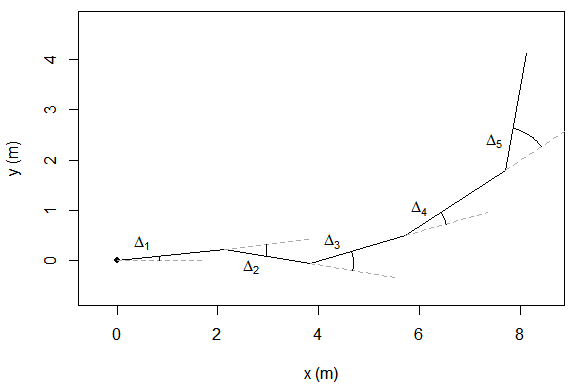A correlated random walk with 5 steps. The direction of step $$i$$ is the direction of step $$(i - 1) + \Delta$$i.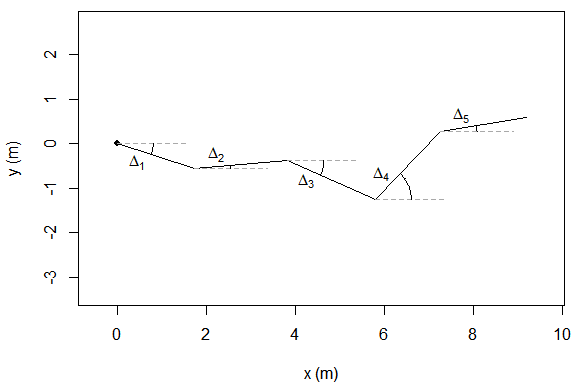A directed walk with 5 steps. The direction of step $$i$$ is the compass direction (here 0$$^\circ$$) + $$\Delta$$i.

Within trajr, trajectories are represented by R objects with class Trajectory. The TrajFromCoords function is used to create a Trajectory object from a set of x-y coordinates, with optional times.

# Define x, y, and time coordinates
coords <- data.frame(x = c(1, 1.5, 2, 2.5, 3, 4),
y = c(0, 0, 1, 1, 2, 1),
times = c(0, 1, 2, 3, 4, 5))
# Create a trajectory from the coordinates
trj <- TrajFromCoords(coords)

# Plot it
plot(trj)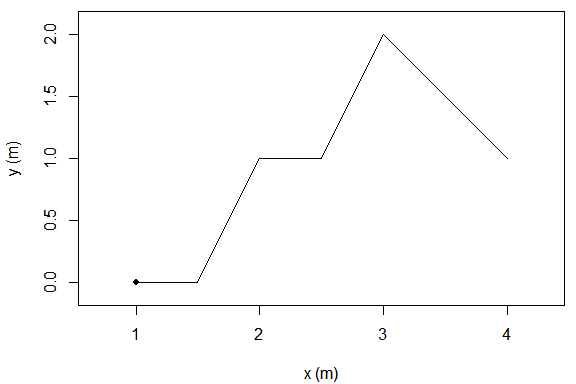Trajectory created from coordinates.

Creating Trajectories

In practice, trajectory coordinates are typically read from a data file such as a CSV file, and then passed in to the TrajFromCoords function.

trj <- TrajFromCoords(coords)

TrajFromCoords assumes that the first column in coords contains x values, the second contains y values, and that points were sampled at a constant sampling frequency of 50 points/second. The help page for TrajFromCoords describes how to alter these defaults - run ?TrajFromCoords to access the help page. Many functions (including TrajFromCoords) contain code examples as part of their help information. You can also display and run an example by calling the example function: i.e. example("TrajFromCoords").

Scaling Trajectories

Trajectories may need to be scaled before analysis. For instance, when a trajectory is digitised from video, the spatial units will be pixels, and require conversion to more meaningful units such as metres. Trajectories are scaled by calling TrajScale, specifying the scale factor and the abbreviation of the transformed units. In the case of a trajectory digitised from a video, you can calculate scale as $$width^{m}/width^{p}$$, where $$width^{m}$$ is the width of an object in metres, and $$width^{p}$$ is the width of the same object in pixels, as measured from the video. A commonly used method to determine the scale is to include a measuring tape in the video, then measure the number of pixels in a length of the tape.

# Example 1
trj <- TrajFromCoords(coords, spatialUnits = "pixels")
# A 10 mm (= .01 m) object had length 47 pixels in the video, scale to metres
trj <- TrajScale(trj, .01 / 47, "m")

# Example 2
# In this video, a 20 cm (= .2 m) object had length 1800 pixels, scale to metres
trj <- TrajScale(trj, .2 / 1800, "m")

Smoothing trajectories

Many trajectories will suffer from noise which may bias the results of some analyses. The TrajSmoothSG function reduces high frequency noise while preserving the shape of the trajectory, by applying a Savitzky-Golay smoothing filter. It requires two arguments (in addition to the trajectory), n, the filter order, and m, the filter length (which must be odd). Refer to the help for the function sgolayfilt (run ?signal::sgolayfilt) for details of the filter function.

There are some issues which should be considered when deciding whether to smooth and by how much. If duplicate points are omitted from a trajectory (i.e. multiple points representing the same stopped location are not explicitly specified), smoothing is likely to result in a segment spanning the gap which is decreasing in velocity, but not stopped. Similarly, smoothing (or too much smoothing) may eliminate short periods of zero (or near-zero) velocity.

trj <- TrajGenerate(200, random = TRUE, angularErrorSd = .25)

# Plot original trajectory
plot(trj, lwd = 1, lty = 1)

# Create a smoothed trajectory, filter order 3, length 31
smoothed <- TrajSmoothSG(trj, p = 3, n = 31)
# Plot it in slightly transparent red
lines(smoothed, col = "#FF0000A0", lwd = 2)

legend("topright", c("Original", "Smoothed"), lwd = c(1, 2), lty = c(1, 1), col = c("black", "red"), inset = 0.01)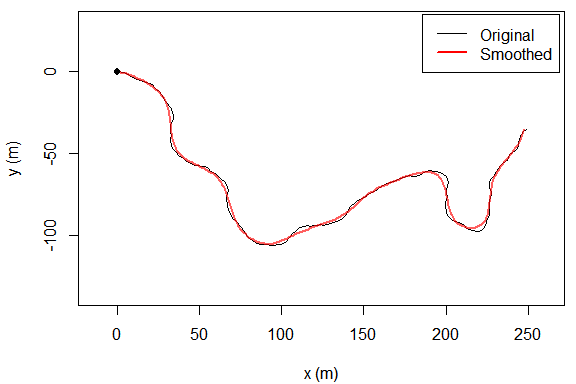Effect of smoothing a trajectory.

Resampling trajectories

trajr provides functions to resample a trajectory to a fixed step length or a fixed step time.

Some functions require a Trajectory with a fixed step length. The process of resampling a trajectory to a fixed step length is called rediscretization. The function TrajRediscretize implements trajectory resampling using the algorithm described by Bovet & Benhamou (1988). There are no clear guidelines for choosing a suitable step length for rediscretization: too small a step length leads to oversampling, leading to high autocorrelation between steps and high variability; too large a step length results in undersampling and loss of information. See Turchin (1998) section 5.2.2 for a discussion.

trj <- TrajGenerate(10, stepLength = 2)

# Plot original trajectory with dots at trajectory coordinates
plot(trj, lwd = 2)
points(trj, draw.start.pt = FALSE, pch = 16, col = "black", cex = 1.2)

# Resample to step length 1
resampled <- TrajRediscretize(trj, 1)

# Plot rediscretized trajectory in red
lines(resampled, col = "#FF0000A0", lwd = 2)
points(resampled, type = 'p', col = "#FF0000A0", pch = 16)
legend("topright", c("Original", "Rediscretized"), col = c("black", "red"),
lwd = 2, inset = c(0.01, 0.02))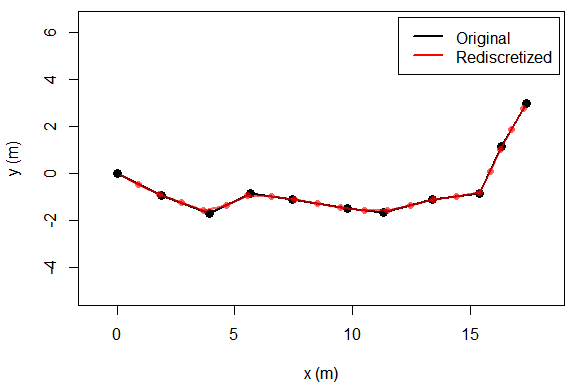Rediscretization of trajectory with $$\mu_{L} = 2$$ to step length $$1$$.

The function TrajResampleTime linearly interpolates points along a trajectory to create a new trajectory with fixed step time intervals.

# Generate trajectory with a point every 2 hours and highly variable speed (which equates to step length)
trj <- TrajGenerate(10, stepLength = 1, fps = .5, timeUnits = "hours", linearErrorSd = .8)

# Plot original trajectory with dots at trajectory coordinates
plot(trj, lwd = 2)
points(trj, draw.start.pt = FALSE, pch = 16, col = "black", cex = 1.2)

# Resample to 1 hourly steps
resampled <- TrajResampleTime(trj, 1)

# Plot rediscretized trajectory in red
lines(resampled, col = "#FF0000A0", lwd = 2)
points(resampled, type = 'p', col = "#FF0000A0", pch = 16)
legend("topright", c("Original", "Resampled"), col = c("black", "red"),
lwd = 2, inset = c(0.01, 0.02))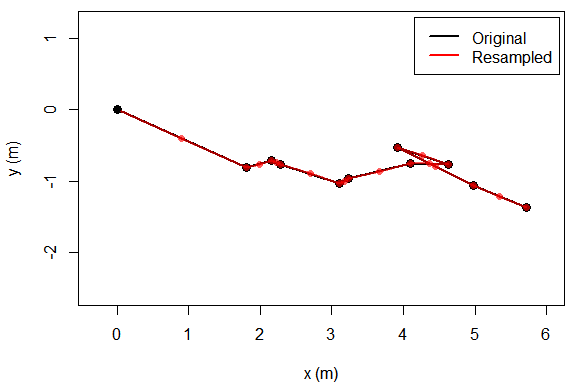Resampling of trajectory with step duration $$1$$ hour.

Other trajectory operations

This table lists trajectory operations not otherwise addressed by this document. Refer to the help for each function for information.

Function Description
TrajRotate Rotates a trajectory
TrajTranslate Translates a trajectory
TrajReverse Reverses a trajectory
TrajGetFPS Returns the frames-per-second of a trajectory
TrajGetNCoords Returns the number of coordinates of a trajectory
TrajGetUnits Returns the spatial units of a trajectory
TrajGetTimeUnits Returns the temporal units of a trajectory
TrajStepLengths Returns the lengths of each step within the trajectory
TrajLength Returns the total length of the trajectory (or a portion)
TrajDistance Returns the straight-line distance from the start to the end of the trajectory (or a portion)
TrajDuration Returns the temporal duration of the trajectory (or a portion)
TrajMeanVelocity Returns the mean velocity vector of the trajectory (or a portion)
TrajAngles Returns the turning angles (radians) of a trajectory
TrajMeanVectorOfTurningAngles Returns the mean vector of the turning angles
TrajExpectedSquareDisplacement Returns the expected square displacement of a correlated random walk

Trajectory analysis

Once you have obtained a correctly scaled Trajectory object, you may call various functions to analyse the trajectory. Broadly speaking, analysis may be divided into measures of speed or acceleration, and measures of straightness or tortuosity.

Analysing speed

The functions TrajVelocity and TrajAcceleration estimate velocity and acceleration as vectors at each point along a trajectory. Velocity is calculated using first-order finite differences, and acceleration uses second-order finite differences. The TrajDerivatives function calculates speed and change in speed along a Trajectory. If the trajectory is noisy, it should be smoothed before the derivatives are calculated (see Smoothing trajectories, but take note of the caveats described there).

trj <- TrajGenerate()

# Smooth before calculating derivatives
smoothed <- TrajSmoothSG(trj, 3, 101)

# Calculate speed and acceleration
derivs <- TrajDerivatives(smoothed)

# Plot change-in-speed and speed
plot(derivs$acceleration ~ derivs$accelerationTimes, type = 'l', col = 'red',
yaxt = 'n',
xlab = 'Time (s)',
ylab = expression(paste('Change in speed (', m/s^2, ')')))
axis(side = 2, col = "red")
lines(derivs$speed ~ derivs$speedTimes, col = 'blue')
axis(side = 4, col = "blue")
mtext('Speed (m/s)', side = 4, line = 3)
abline(h = 0, col = 'lightGrey')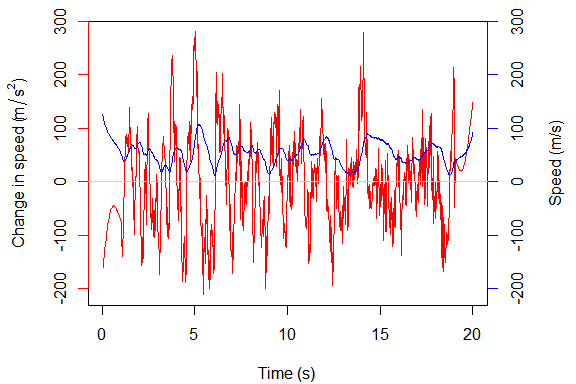Speed and acceleration over time.

Once the trajectory speed has been obtained, it is simple to calculate values such as mean, maximum, minimum or standard deviation of speed: mean(derivs$speed), max(derivs$speed), min(derivs$speed), or sd(derivs$speed). Similarly, calculations excluding periods when speed drops below (or above) some value are also straightforward, for example: mean(derivs$speed[deriv$speed > STOPPED_SPEED]). In addition, the function TrajSpeedIntervals allows you to determine the time intervals within the trajectory that satisfy a speed constraint, either slower than some speed, faster than a speed, or both. This may be useful, for example, when testing how often, or for how long, a flying insect hovers, or a running insect stops, i.e. when its speed drops below some threshold. The object returned by a call to TrajSpeedIntervals can be plotted.

# Simulate a flying bee
trj <- TrajGenerate(100, angularErrorSd = 0.3, stepLength = 0.001)
# Smooth the trajectory
trj <- TrajSmoothSG(trj, 3, 51)

# Calculate hovering intervals
intervals <- TrajSpeedIntervals(trj, slowerThan = 2)
print(intervals)
#>   startFrame startTime stopFrame  stopTime  duration
#> 1         11 0.2337088        27 0.5488033 0.3150945
#> 2         85 1.7073675        94 1.8866781 0.1793107
# Plot speed over time with hovering intervals highlighted
plot(intervals)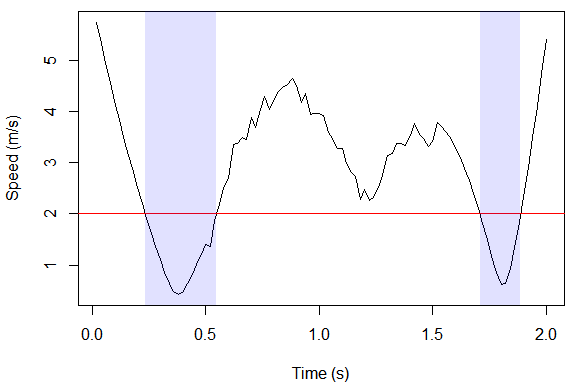Speed over time, hovering intervals (speed < 2 m/s) highlighted.

Analysing straightness

Straightness index

Various methods to measure the straightness, or conversely, tortuosity, of trajectories are available within trajr. The simplest is $$D/L$$, where $$D$$ is the distance from the start to the end of the trajectory, and $$L$$ is the length of the trajectory. This straightness index is calculated by the function TrajStraightness, and is a number ranging from 0 to 1, where 1 indicates a straight line. Benhamou (2004) considers the straightness index to be a reliable measure of the efficiency of a directed walk, but inapplicable to random trajectories. Batschelet (1981) considers this straightness index to be an approximation of r, which is the length of the mean vector of turning angles after rediscretizing to a constant step length. r can be calculated by calling Mod(TrajMeanVectorOfTurningAngles(trj)), assuming trj is a Trajectory with constant step length.

Within this section, most of the figures show the appropriate statistic plotted for multiple randomly generated correlated random walks which vary in the standard deviations of angular errors, $$\sigma_{\Delta}$$ (higher values of $$\sigma_{\Delta}$$ produce more tortuous trajectories).

# Generate some trajectories for use in examples
n <- 100
# Random magnitude of angular errors
angularErrorSd <- runif(n, 0, 2)

# Generate some trajectories with varying angular errors
trjs <- lapply(1:n, function(i) TrajGenerate(500, stepLength = 2,
angularErrorSd = angularErrorSd[i]))

# Rediscretize each trajectory to a range of step sizes
stepSizes <- c(1, 2, 10)
reds <- lapply(stepSizes, function(ss) lapply(1:n, function(i) TrajRediscretize(trjs[[i]], ss)))
# Calculate straightness (D/L) for all of the rediscretized trajectories
ds <- sapply(reds, function(rtrjs) sapply(1:n, function(i) TrajStraightness(rtrjs[[i]])))
# Calculate alternate straightness (r) for all of the rediscretized trajectories
rs <- sapply(reds, function(rtrjs) sapply(1:n, function(i) Mod(TrajMeanVectorOfTurningAngles(rtrjs[[i]]))))

# Plot both indices on the same graph
plot(rep(angularErrorSd, 3), rs,
pch = 16, cex = .8,
col = c(rep('red', n), rep('blue', n), rep('darkgreen', n)),
xlab = expression(sigma[Delta]), ylab = "Straightness",
ylim = range(c(ds, rs)))
points(rep(angularErrorSd, 3), ds,
pch = 3, cex = .8,
col = c(rep('red', n), rep('blue', n), rep('darkgreen', n)))

legend("bottomleft", c(expression(italic(r)), "D/L", paste("Step length", stepSizes)),
pch = c(16, 3, 16, 16),
col = c("black", "black", "red", "blue", "darkgreen"), inset = 0.01)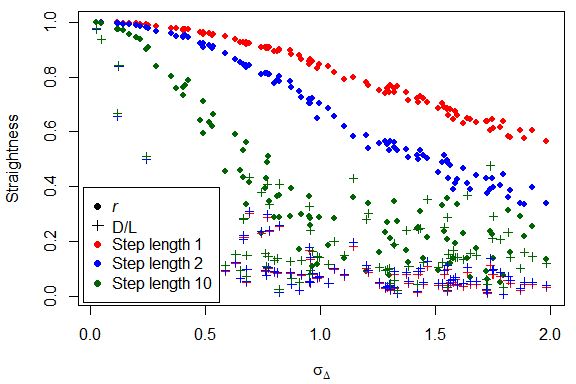Two straightness indices (r, dots, and D/L, crosses) as a function of $$\sigma_{\Delta}$$, plotted for several different step sizes (linear axes).

Sinuosity

The sinuosity index defined by Benhamou (2004) may be an appropriate measure of the tortuosity of a random search path. Sinuosity is a function of the mean cosine of turning angles, and is a corrected form of the original sinuosity index defined by Bovet & Benhamou (1988). The function TrajSinuosity2 calculates the corrected form while TrajSinuosity calculates the original index. In general, TrajSinuosity2 should be used rather than TrajSinuosity. The uncorrected form of sinuosity (TrajSinuosity1) should be calculated for a trajectory with a constant step length, so trajectories may first require rediscretization. In the absence of a biologically meaningful step size, rediscretizing to the mean step length of the trajectory will produce a trajectory with approximately the same shape and number of steps as the original.

# Calculate sinuosity for all of the rediscretized trajectories
sins <- sapply(reds, function(rtrjs) sapply(1:n, function(i) TrajSinuosity2(rtrjs[[i]])))

# Plot sinuosity vs angular error
plot(rep(angularErrorSd, 3), sins,
pch = 16, cex = .8,
col = c(rep('red', n), rep('blue', n), rep('darkgreen', n)),
xlab = expression(sigma["angular error"]), ylab = "Sinuosity")

legend("bottomright", paste("Step length", stepSizes),
pch = 16, col = c("red", "blue", "darkgreen"), inset = 0.01)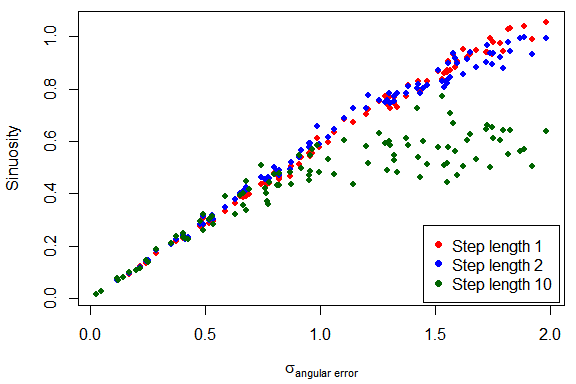Sinuosity as a function of $$\sigma_{\Delta}$$, plotted for several different step sizes (linear axes).

Emax

$$E^{a}_{max}$$ is a dimensionless estimate of the maximum expected displacement of a trajectory. Larger $$E^{a}_{max}$$ values (approaching infinity) represent straighter paths (Cheung, Zhang, Stricker, & Srinivasan, 2007). $$E^{b}_{max}$$ is $$E^{a}_{max}$$ multiplied by the mean step length, so gives the maximum possible displacement in spatial units. TrajEmax returns the value of $$E^{a}_{max}$$ for a trajectory, or $$E^{b}_{max}$$ if the argument eMaxB is TRUE. TrajEmax differentiates between random and directed walks; see ?TrajEmax for details.

# Calculate Emax for all of the rediscretized trajectories (from the previous example)
emaxs <- sapply(reds, function(rtrjs) sapply(1:n, function(i) TrajEmax(rtrjs[[i]])))
emaxs[emaxs < 0] <- NA # Avoid warnings when plotting on log axes

# Plot Emax vs angular error on log axes
plot(rep(angularErrorSd, 3), emaxs,
log = 'xy',
pch = 16, cex = .8,
col = c(rep('red', n), rep('blue', n), rep('darkgreen', n)),
xlab = expression(sigma["angular error"]), ylab = expression(E[max]))

legend("bottomleft", c("Step length 1", "Step length 2", "Step length 10"),
pch = 16, col = c("red", "blue", "darkgreen"), inset = 0.01)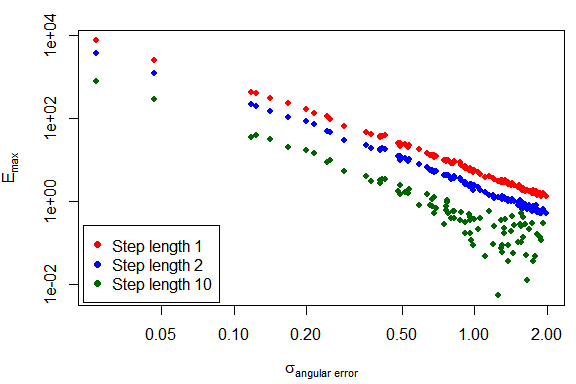Emax as a function of $$\sigma_{\Delta}$$ (logarithmic axes).

Fractal dimension

Fractal dimension has been considered a promising measure of straightness or tortuosity, varying between 1 (straight line movement) and 2 (Brownian motion). However, several studies have found it inappropriate for use with animal trajectories, as animal trajectories are not fractal curves, and the fractal dimension of a non-fractal curve depends critically on the range of step sizes used to calculate it (Nams, 2006; Turchin, 1996). Nonetheless, fractal dimension continues to be used, and the TrajFractalDimension function, by default, calculates fractal dimension using the modified dividers method to account for truncation error (Nams, 2006). See ?TrajFractalDimension and ?TrajFractalDimensionValues for more information.

# Use the same range of step sizes for all trajectories
stepSizes <- TrajLogSequence(.1, 7, 10)

# Fractal dimension is a slow calculation, so just plot a subset
# of trajectories from the previous example
fn <- n / 4
use <- sample.int(fn, n = length(angularErrorSd))
fangularErrorSd <- angularErrorSd[use]

# Calculate fractal dimension for all of the rediscretized trajectories
d <- sapply(reds, function(rtrjs) sapply(use, function(i) {
TrajFractalDimension(rtrjs[[i]], stepSizes)
}))

# Plot fractal dimension vs angular error
plot(rep(fangularErrorSd, 3), d,
pch = 16, cex = .8,
col = c(rep('red', fn), rep('blue', fn), rep('darkgreen', fn)),
xlab = expression(sigma["angular error"]), ylab = "Fractal dimension")

legend("topleft", c("Step length 1", "Step length 2", "Step length 10"),
pch = 16, col = c("red", "blue", "darkgreen"), inset = 0.01)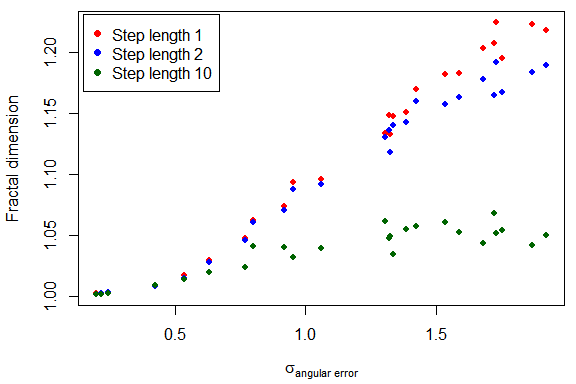Fractal dimension as a function of $$\sigma_{\Delta}$$.

Directional change (DC and SDDC)

Directional change is defined as the change in direction over time, and has been used to assess locomotor mimicry in butterflies (Kitamura & Imafuku, 2015). Directional change is defined for each pair of steps, so a trajectory (or a portion thereof) may be characterised by the mean (DC) and standard deviation (SDDC) of all directional changes. DC may be used as an index of nonlinearity, and SDDC as a measure of irregularity. The directional changes for a trajectory are calculated by the function TrajDirectionalChange, so SD may be calculated as mean(TrajDirectionalChange(trj)), and SDDC as sd(TrajDirectionalChange(trj)). Note that directional change cannot be calculated on rediscretized trajectories, as rediscretizing discards time information.

# Calculate DC and SDDC for all of the original trajectories (from above)
dcs <- sapply(1:n, function(i) mean(TrajDirectionalChange(trjs[[i]])))
sddcs <- sapply(1:n, function(i) sd(TrajDirectionalChange(trjs[[i]])))

# Plot DC vs angular error
plot(angularErrorSd, dcs,
pch = 16, cex = .8, col = "blue",
xlab = expression(sigma["angular error"]), ylab = "DC")
points(angularErrorSd, sddcs, pch = 16, cex = .8, col = 'red')
legend("bottomright", c("DC", "SDDC"),
pch = 16, col = c("blue", "red"), inset = 0.01)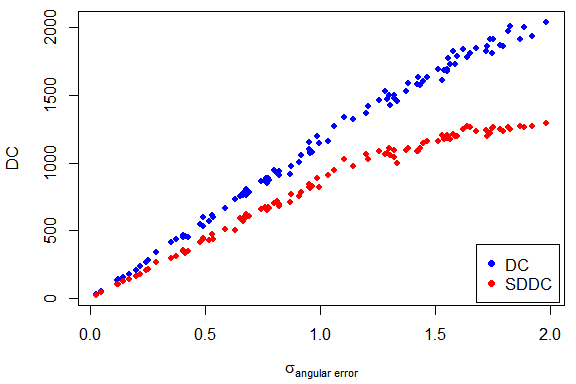DC and SDDC as a function of $$\sigma_{\Delta}$$.

Direction autocorrelation

The direction autocorrelation function was devised to detect and quantify regularities within trajectories, in the context of locomotor mimicry by ant-mimics (Shamble, Hoy, Cohen, & Beatus, 2017). It detects wave-like periodicities, and provides an indication of their wavelength and amplitude, so can be used to detect, for example, the winding movements of trail-following ants. The function $$C(\Delta s)$$ is applied to a rediscretized trajectory, and calculates the differences in step angles at all steps separated by $$\Delta$$, for a range of $$\Delta s$$. The position of the first local minimum in $$C(\Delta s)$$ (i.e. the 2-dimensional position $$(\Delta s, c(\Delta s))$$) may be used to characterise the periodicity within a trajectory.

The function is calculated by TrajDirectionAutocorrelations, and the result may be plotted. The position of the first local minimum (or maximum) is calculated by TrajDAFindFirstMinimum (or TrajDAFindFirstMaximum). Some trajectories will not have a first local minimum (or maximum), which indicates that they do not contain regular changes in direction.

trj <- TrajGenerate(1000)
corr <- TrajDirectionAutocorrelations(trj)
plot(corr)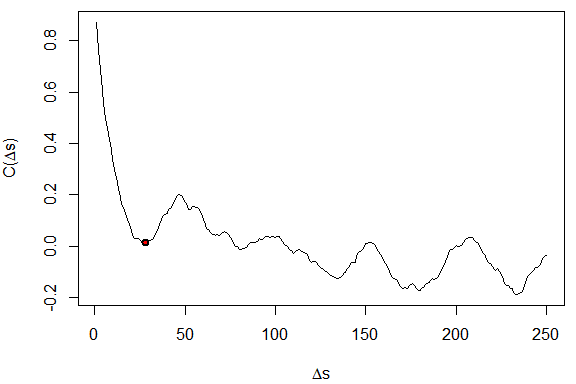Direction autocorrelation of a random trajectory with first local minimum indicated.

Working with multiple trajectories

Building multiple trajectories

As trajectory studies are likely to involve multiple trajectories per individual, per species, or trajectories for multiple species, trajr provides some functions to simplify the manipulation of multiple trajectories. TrajsBuild assumes that you have a set of trajectory files (such as CSV files), each of which may have a scale and a frames-per-second value. The function reads each of the files specified in a list of file names, optionally using a custom function, then passes the result to TrajFromCoords, which assumes that the first column is x, the second is y, and there is no time column. If these assumptions are incorrect, the csvStruct argument can be specified to identify the x, y and time columns (see the example below). TrajsBuild optionally scales the trajectories with the specified scale (by calling TrajScale), and optionally smooths them with the specified smoothing parameters (by calling TrajSmoothSG). The value returned is a list of Trajectory objects.

tracks <- as.data.frame(rbind(
c("3527.csv", "Zodariid2 sp1",     "spider",       "red"),
c("3530.csv", "Daerlac nigricans", "mimic bug",    "blue"),
c("3534.csv", "Daerlac nigricans", "mimic bug",    "blue"),
c("3537.csv", "M. erythrocephala", "mimic spider", "blue"),
c("3542.csv", "Polyrhachis sp1",   "ant",          "black"),
c("3543.csv", "Polyrhachis sp1",   "ant",          "black"),
c("3548.csv", "Crematogaster sp1", "ant",          "black")
), stringsAsFactors = FALSE)
colnames(tracks) <- c("filename", "species", "category", "col")
# If your list is read from a CSV file which contains empty lines,
# remove them like this:
tracks <- na.omit(tracks)
# Order of columns in the CSV files is unknown so identify them by name
csvStruct <- list(x = "x", y = "y", time = "Time")
sd_speed <- sd(derivs$speed) # Measures of straightness sinuosity <- TrajSinuosity(trj) resampled <- TrajRediscretize(trj, .001) Emax <- TrajEmax(resampled) # Periodicity corr <- TrajDirectionAutocorrelations(resampled, 60) first_min <- TrajDAFindFirstMinimum(corr) # Return a list with all of the statistics for this trajectory list(mean_speed = mean_speed, sd_speed = sd_speed, sinuosity = sinuosity, Emax = Emax, min_deltaS = first_min, min_C = first_min ) } # Calculate all stats for trajectories in the list # which was built in the previous example stats <- TrajsMergeStats(trjs, characteriseTrajectory) print(stats) #> mean_speed sd_speed sinuosity Emax min_deltaS min_C #> deltaS 0.061296301 0.009069424 0.9833243 3180.72321 33 0.899013229 #> 2 0.010573501 0.005718966 20.1799859 100.43632 50 0.303049396 #> 3 0.014175447 0.005206138 10.5781944 692.21342 NA NA #> 4 0.006245555 0.003005911 6.3442130 67.52479 7 0.930992488 #> 5 0.005344287 0.007936181 60.5565802 14.90601 NA NA #> 6 0.019378799 0.005363627 3.3069095 74.33277 35 0.002917922 #> 7 0.014796829 0.002573456 2.4142475 112.93798 NA NA PCA analysis of multiple trajectories Principal components analysis (PCA) is a commonly used statistical technique which can be used to visualise similarities and differences between groups of trajectories. In R, the function prcomp is usually used to perform a PCA. Prcomp will not process data containing NAs (in R, the value NA is used to indicate a ‘Not Available’, or missing, value). However, NAs can be potentially informative, especially when using the first local minimum of the direction autocorrelation function - in this case, an NA indicates that there was no periodicity detected in the trajectory. To avoid discarding this information, Trajr provides a utility function, TrajsStatsReplaceNAs. It is used to replace NA values with some other value, and optionally adds an additional column to the data which flags trajectories which originally contained NA values. It operates on a single column at a time. #--- # Custom PCA plotting function customPcaPlot <- function(x, xlabs, xcols, choices = 1L:2L, ycol = "#ff2222aa", ...) { # Draw points pts <- t(t(x$x[, choices]))
plot(pts, type = "p",
xlim = extendrange(pts[, 1L]), ylim = extendrange(pts[, 2L]),
asp = 1,
xlab = "PC1", ylab = "PC2", pch = 16, col = xcols, ...)
text(pts, labels = xlabs, pos = 1, ...)

# Draw arrows
axs <- t(t(x$rotation[, choices])) * 3.5 text(axs, labels = dimnames(axs)[[1L]], col = ycol, ...) arrows(0, 0, axs[, 1L] * .8, axs[, 2L] * .8, length = .1, col = ycol) } # --- # Here we are operating on the statistics from the previous example # Get rid of NAs in stats because prcomp can't handle them. # First fix min_deltaS, and add an extra column which flags non-periodic trajectories pcaStats <- TrajsStatsReplaceNAs(stats, "min_deltaS", replacementValue = 2 * max(stats$min_deltaS, na.rm = TRUE),
flagColumn = "no_first_min")
# Also get rid of NAs in min_C - no need to add another column since it would duplicate no_first_min
pcaStats <- TrajsStatsReplaceNAs(pcaStats, "min_C",
replacementValue = 2 * max(stats$min_C, na.rm = TRUE)) # Perform the PCA PCA <- prcomp(pcaStats, scale. = TRUE) # Plot it using custom plotting function. Could just call biplot instead customPcaPlot(PCA, tracks$category, tracks$col, cex = .8) legend("bottomleft", c("Spider", "Mimic", "Ant"), pch = 16, col = c('red', 'blue', 'black'), inset = c(0.01, .02))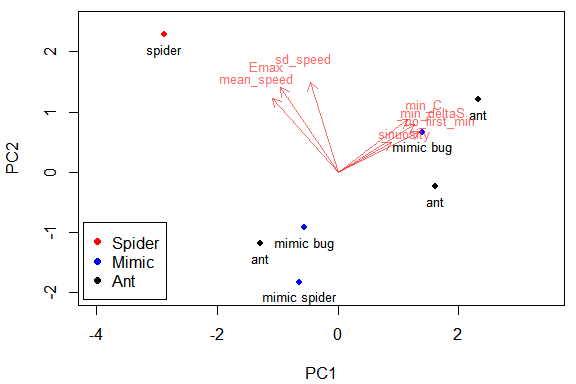PCA of trajectory characterisations. The plot above shows that the trajectory of the non-mimetic spider (red dot) seems quite different from the trajectories of ant mimicking bugs, ant-mimicking spiders (blue dots) and ants (black dots). The arrows indicate the importance and direction of the parameters that separate the trajectories. From the plot, it seems that the non-mimetic spider varies mainly in speed, (it is faster and more variable) and Emax (it walks in a straighter path). While these trajectories have been digitised from videos of walking animals, clearly this example does not contain enough data to be meaningful. Other operations The function TrajsStepLengths returns a vector containing all of the step lengths in a list of trajectories. summary(TrajsStepLengths(trjs)) #> Min. 1st Qu. Median Mean 3rd Qu. Max. #> 1.000e-10 7.813e-05 2.266e-04 2.266e-04 3.243e-04 1.550e-03 TrajConvertTime is a convenience function that converts a delimited time string to a numeric value. This may be useful if your data contains times which are formatted as strings with millisecond accuracy. In general, the base R function strptime should be used to convert strings to times, but it does not handle milliseconds. t <- data.frame(time = c("0:00:00:029", "0:01:00:216", "0:02:01:062", "1:00:02:195", "1:06:03:949", "1:42:04:087"), stringsAsFactors = FALSE) t$seconds <- TrajConvertTime(t\$time)
t
#>          time  seconds
#> 1 0:00:00:029    0.029
#> 2 0:01:00:216   60.216
#> 3 0:02:01:062  121.062
#> 4 1:00:02:195 3602.195
#> 5 1:06:03:949 3963.949
#> 6 1:42:04:087 6124.087

Random trajectory generation

Trajr allows you to generate random trajectories with the TrajGenerate function. Random trajectories may be used in simulation studies, or simply for experimenting with trajectory analysis, and it has been used to generate the majority of trajectories in this document. By default, TrajGenerate returns a correlated random walk, however, by specifying different arguments, a variety of trajectory types can be created.

# Correlated random walk
trj <- TrajGenerate(n = 200)
# Plot it
plot(trj)
mtext("a)", 3, -1.3, adj = .05)

# Directed walk
trj <- TrajGenerate(n = 20, random = FALSE)
plot(trj)
mtext("b)", 3, -1.3, adj = .05)

# Brownian motion
trj <- TrajGenerate(n = 500, angularErrorDist = function(n) stats::runif(n, -pi, pi))
plot(trj)
mtext("c)", 3, -1.3, adj = .05)

# Levy walk - path lengths follow a Cauchy distribution
trj <- TrajGenerate(linearErrorDist = stats::rcauchy)
plot(trj)
mtext("d)", 3, -1.3, adj = .05)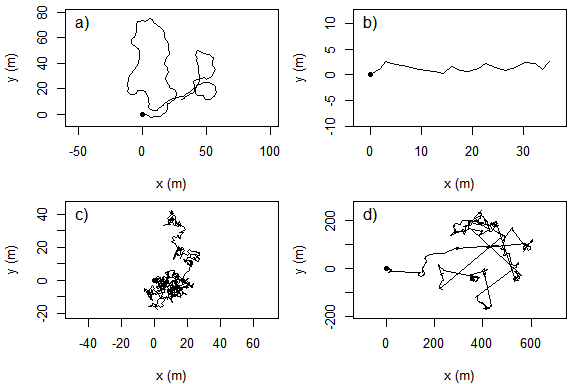Some generated trajectories. a) correlated walk, b) directed walk, c) brownian motion, d) Levy walk.

trajr provides limited assistance for building more sophisticated heterogeneous trajectories. Functions exist for splitting (TrajSplit) and merging (TrajMerge) trajectories, for detecting when trajectories cross polygon boundaries (TrajInPolygon) and splitting a trajectory at a polygon crossing (TrajSplitAtFirstCrossing).

Performance

Processing time can be a problem when operating on large numbers of large trajectories.

• Use an appropriate step length for rediscretization. Too small a step length will result in trajectories with an excessive number of steps and will take a long time to process.
• Some steps, such as calculating directional autocorrelations, may be amenable to memoisation.

And finally…

Trajr is open source, with the source available on Github. If you have any problems or suggestions, email .